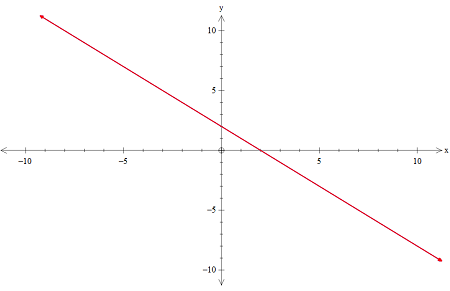# Solve the system by graphing. (Enter your answers as a comma-separated list. If the system is...

## Question:

Solve the system by graphing. (Enter your answers as a comma-separated list. If the system is inconsistent, enter INCONSISTENT. If the system is dependent, enter DEPENDENT.)

x + y = 2

-x - y = -2

## Consistent System of Equations:

The system of equations given is said to be consistent if they have at least one solution by solving them simultaneously whereas the system of equations is said to be dependent if they have infinitely many feasible solutions.A system is said to be consistent if it has at least one solution and this system of equations will have many solutions as both the lines are overlapping.

If both the system of equations have infinite solutions, the system is said to be dependent.

Thus, the system of equations provided to us is CONSISTENT and DEPENDENT